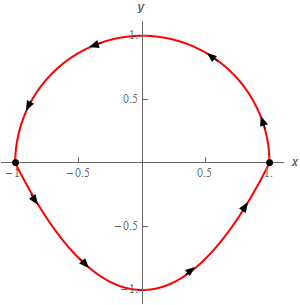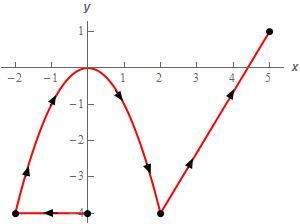Paul's Online Notes
Home / Calculus III / Line Integrals / Line Integrals of Vector Fields
Show Mobile Notice Show All Notes Hide All Notes
Mobile Notice
You appear to be on a device with a "narrow" screen width (i.e. you are probably on a mobile phone). Due to the nature of the mathematics on this site it is best views in landscape mode. If your device is not in landscape mode many of the equations will run off the side of your device (should be able to scroll to see them) and some of the menu items will be cut off due to the narrow screen width.

### Section 16.4 : Line Integrals of Vector Fields

1. Evaluate $$\displaystyle \int\limits_{C}{{\vec F\centerdot d\vec r}}$$ where $$\vec F\left( {x,y} \right) = {y^2}\,\vec i + \left( {3x - 6y} \right)\vec j$$ and $$C$$ is the line segment from $$\left( {3,7} \right)$$ to $$\left( {0,12} \right)$$. Solution
2. Evaluate $$\displaystyle \int\limits_{C}{{\vec F\centerdot d\vec r}}$$ where $$\vec F\left( {x,y} \right) = \left( {x + y} \right)\,\vec i + \left( {1 - x} \right)\vec j$$ and $$C$$ is the portion of $$\displaystyle\frac{{{x^2}}}{4} + \frac{{{y^2}}}{9} = 1$$ that is in the 4th quadrant with the counter clockwise rotation. Solution
3. Evaluate $$\displaystyle \int\limits_{C}{{\vec F\centerdot d\vec r}}$$ where $$\vec F\left( {x,y} \right) = {y^2}\,\vec i + \left( {{x^2} - 4} \right)\vec j$$ and $$C$$ is the portion of $$y = {\left( {x - 1} \right)^2}$$ from $$x = 0$$ to $$x = 3$$. Solution
4. Evaluate $$\displaystyle \int\limits_{C}{{\vec F\centerdot d\vec r}}$$ where $$\vec F\left( {x,y,z} \right) = {{\bf{e}}^{2x}}\,\vec i + z\left( {y + 1} \right)\vec j + {z^3}\,\vec k$$ and $$C$$ is given by $$\vec r\left( t \right) = {t^3}\,\vec i + \left( {1 - 3t} \right)\vec j + {{\bf{e}}^t}\,\vec k$$ for $$0 \le t \le 2$$. Solution
5. Evaluate $$\displaystyle \int\limits_{C}{{\vec F\centerdot d\vec r}}$$ where $$\vec F\left( {x,y} \right) = 3y\,\vec i + \left( {{x^2} - y} \right)\vec j$$ and $$C$$ is the upper half of the circle centered at the origin of radius 1 with counter clockwise rotation and the portion of $$y = {x^2} - 1$$ from $$x = - 1$$ to $$x = 1$$. See the sketch below.Solution
6. Evaluate $$\displaystyle \int\limits_{C}{{\vec F\centerdot d\vec r}}$$ where $$\vec F\left( {x,y} \right) = xy\,\vec i + \left( {1 + 3y} \right)\vec j$$ and $$C$$ is the line segment from $$\left( {0, - 4} \right)$$ to $$\left( { - 2, - 4} \right)$$ followed by portion of $$y = - {x^2}$$ from $$x = - 2$$ to $$x = 2$$ which is in turn followed by the line segment from $$\left( {2, - 4} \right)$$ to $$\left( {5,1} \right)$$. See the sketch below.Solution
7. Evaluate $$\displaystyle \int\limits_{C}{{\vec F\centerdot d\vec r}}$$ where $$\vec F\left( {x,y} \right) = \left( {6x - 2y} \right)\,\vec i + {x^2}\vec j$$ for each of the following curves.
1. $$C$$ is the line segment from $$\left( {6, - 3} \right)$$ to $$\left( {0,0} \right)$$ followed by the line segment from $$\left( {0,0} \right)$$ to $$\left( {6,3} \right)$$.
2. $$C$$ is the line segment from $$\left( {6, - 3} \right)$$ to $$\left( {6,3} \right)$$.
Solution
8. Evaluate $$\displaystyle \int\limits_{C}{{\vec F\centerdot d\vec r}}$$ where $$\vec F\left( {x,y} \right) = 3\,\vec i + \left( {xy - 2x} \right)\vec j$$ for each of the following curves.
1. $$C$$ is the upper half of the circle centered at the origin of radius 4 with counter clockwise rotation.
2. $$C$$ is the upper half of the circle centered at the origin of radius 4 with clockwise rotation.
Solution